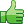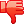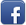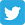# Auto-populating dropdowns and multiple forms.

Posted on 16th Feb 2014 07:03 pm by admin

Here's what I have so far:

First drop down = select a state (works)
This populates the second drop down (works)
Second drop down = select a city (works)
This populates the third drop down with local restaurant names (not quite)

When I select a restaurant from the drop down the form is submitted but the \$rest_name variable isn't carried over

Site: www.abcgreatdeals.com
Select:California, La Quinta for an example

I'm sure it's something simple that I'm just over looking. Thanks for any input.

Code: [Select]<form id="form1" name="form1" method="post" action="<?php echo \$PHP_SELF; ?>" enctype="multipart/form-data">
<?php
if (isset (\$_POST['state']))
{
if (\$state == '99')
{
?>
<script type="text/javascript">
<!--
window.location = "http://www.abcgreatdeals.com"
//-->
</script>
<?php
}
?>
<select name="state" CLASS="formTextbox" onchange="form1.submit();">
<option value="<?=\$state;?>"><?=\$state;?></option>
<option value="99">Start Over</option>
<?php
}
else{
\$Get_State = mysql_query("SELECT DISTINCT state FROM coupons ORDER BY state",\$db_link);
?>
<select name="state" CLASS="formTextbox" onchange="this.form.submit();">
<OPTION SELECTED VALUE="">Select A State</OPTION>
<? while(\$get_it = mysql_fetch_array(\$Get_State)) { ?>
<option value="<?=\$get_it['state'];?>"><?=\$get_it['state'];?></option>
<?
}}
if (isset (\$_POST['state']))
{?>
</select>
</form>
<form id="form2" name="form2" method="post" action="<?php echo \$PHP_SELF; ?>" enctype="multipart/form-data">
<?php
if (isset (\$_POST['city']) != '')
{
if (\$city == '99')
{
?>
<script type="text/javascript">
<!--
window.location = "http://www.abcgreatdeals.com"
//-->
</script>
<?php
}
?>
<input type="hidden" name="state" value="<?="\$state"; ?>">
<select name="city" CLASS="formTextbox" onchange="form2.submit();">
<option value="<?=\$city;?>"><?=\$city;?></option>
<option value="99">Start Over</option>
<?php
}
else{
\$Got_City = mysql_query("SELECT DISTINCT city FROM coupons WHERE state='\$state' ORDER BY city",\$db_link);
?>
<input type="hidden" name="state" value="<?="\$state"; ?>">
<select name="city" CLASS="formTextbox" onchange="form2.submit();">
<OPTION SELECTED VALUE="">Select A City</OPTION>
<? while(\$got_it = mysql_fetch_array(\$Got_City)) { ?>
<?
if (\$got_it['city'] != ''){ ?>
<option value="<?=\$got_it['city'];?>"><?=\$got_it['city'];?></option>
<?
}
else { ?>
<option value="99">Start Over</option>
<? } ?>
<?
}}
if (isset (\$_POST['city']))
{?>
</select>
</form>
<form id="form3" name="form3" method="post" action="<?php echo \$PHP_SELF; ?>" enctype="multipart/form-data">
<?php
if (isset (\$_POST['rest']) != '')
{
if (\$rest == '99')
{
?>
<script type="text/javascript">
<!--
window.location = "http://www.abcgreatdeals.com"
//-->
</script>
<?php
}
?>
<input type="hidden" name="city" value="<?="\$city"; ?>">
<input type="hidden" name="state" value="<?="\$state"; ?>">
<select name="rest" CLASS="formTextbox" onchange="form3.submit();">
<option value="<?=\$rest_name;?>"><?=\$rest_name?></option>
<option value="99">Start Over</option>
<?php
}
else{
\$Get_Rest = mysql_query("SELECT DISTINCT rest_name FROM coupons WHERE state='\$state' AND city='\$city'",\$db_link);
?>
<input type="hidden" name="state" value="<?=\$state?>">
<input type="hidden" name="city" value="<?=\$city?>">
<select name="rest" CLASS="formTextbox" onchange="form3.submit();">
<OPTION SELECTED VALUE="">Select A Restaurant</OPTION>
<?
while(\$got_rest = mysql_fetch_array(\$Get_Rest)){
\$rest_name = stripslashes(\$got_rest['rest_name']);
?>
<option value="<?=\$rest_name;?>"><?=\$rest_name?></option>
<?
}}}}
if (isset (\$_POST['rest']))
{?>
</select>
</form>
<?php
if (\$rest_name){
echo "<strong>Click on coupons to print individually</strong>

";
}
if (\$rest_name)
{
\$Get_Coupons = mysql_query("SELECT * FROM coupons WHERE rest_name='\$rest_name' ORDER BY coupon_rank DESC",\$db_link);

8320##### Other forums

How to use Ajax to verify data on a DB ?
Hello everyone, well i want to know how to check a value if it exists or not on a Data base and capt

private constructor
Hello,

Can we create a constructor as private? If yes, what is the use of it? If no, why can'

split string
Hi all

i have some names (imploded by comma):
Now, i would